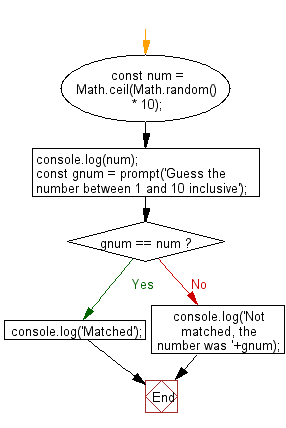# JavaScript: Display a message when a number is between a range

## JavaScript Basic: Exercise-8 with Solution

Write a JavaScript program where the program takes a random integer between 1 to 10, the user is then prompted to input a guess number. If the user input matches with guess number, the program will display a message "Good Work" otherwise display a message "Not matched"

Sample Solution:

HTML Code:

``````<!DOCTYPE html>
<html>
<meta charset=utf-8 />
<title>Guess a number</title>
<body>
</body>
</html>
```
```

JavaScript Code:

``````// Get a random integer from 1 to 10 inclusive
const num = Math.ceil(Math.random() * 10);
console.log(num);
const gnum = prompt('Guess the number between 1 and 10 inclusive');
if (gnum == num)
console.log('Matched');
else
console.log('Not matched, the number was '+gnum);
```
```

Sample Output:

```Matched
```

Explanation:

The Math.ceil() function is used to get the smallest integer greater than or equal to a given number.

The Math.random() function is used to get a floating-point, pseudo-random number in the range [0, 1] that is, from 0 (inclusive) up to but not including 1 (exclusive), which you can then scale to your desired range.

Flowchart:ES6 Version:

``````// Get a random integer from 1 to 10 inclusive
var num = Math.ceil(Math.random() * 10);
console.log(num);
var gnum = prompt('Guess the number between 1 and 10 inclusive');
if (gnum == num) console.log('Matched');else console.log('Not matched, the number was ' + gnum);
``````

Live Demo:

See the Pen JavaScript basic animation - basic-ex-8 by w3resource (@w3resource) on CodePen.

Improve this sample solution and post your code through Disqus

What is the difficulty level of this exercise?

Test your Programming skills with w3resource's quiz.

﻿

## JavaScript: Tips of the Day

Converts a string to title case

Example:

```const toTitleCase = str =>
str
.match(/[A-Z]{2,}(?=[A-Z][a-z]+[0-9]*|\b)|[A-Z]?[a-z]+[0-9]*|[A-Z]|[0-9]+/g)
.map(x => x.charAt(0).toUpperCase() + x.slice(1))
.join(' ');
console.log(toTitleCase('some_database_field_name')); // 'Some Database Field Name'
console.log(toTitleCase('Some label that needs to be title-cased')); // 'Some Label That Needs To Be Title Cased'
console.log(toTitleCase('some-package-name')); // 'Some Package Name'
console.log(toTitleCase('some-mixed_string with spaces_underscores-and-hyphens')); // 'Some Mixed String With Spaces Underscores And Hyphens'
```

Output:

```"Some Database Field Name"
"Some Label That Needs To Be Title Cased"
"Some Package Name"
"Some Mixed String With Spaces Underscores And Hyphens"
```